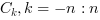Scilab Home page | Wiki | Bug tracker | Forge | Mailing list archives | ATOMS | File exchange
Change language to: Français - Português - 日本語 - Русский

See the recommended documentation of this function

Scilab Help >> Signal Processing > xcorr

xcorr

Computes discrete auto or cross correlation

Calling Sequence

[c [,lagindex]] = xcorr(x [,maxlags [,scaling]])
[c [,lagindex]] = xcorr(x,y [,maxlags [,scaling]])

Parameters

x

a vector of real or complex floating point numbers.

y

a vector of real or complex floating point numbers. The default value is x.

maxlags

a scalar with integer value greater than 1. The default value is n. Where n is the maximum of the x and y vector length.

scaling

a character string with possible value: "biased", "unbiased", "coeff", "none". The default value is "none".

c

a vector of real or complex floating point numbers with same orientation as x.

lagindex

a row vector, containing the lags index corresponding to the c values.

Description

• c=xcorr(x) computes the un-normalized discrete auto correlation:and return in c the sequence of auto correlation lagswith n is the length of x
• xcorr(x,y) computes the un-normalized discrete cross correlation:and return in c the sequence of auto correlation lagswith n is the maximum of x and y length's.

If the maxlags argument is given xcorr returns in c the sequence of auto correlation lags. If maxlags is greater than length(x), the first and last values of c are zero.

The scaling argument decribes howis normalized before being returned in c:

• "biased":c=/n.
• "unbiased":c=./(n-(-maxlags:maxlags)).
• "coeff":c=/(norm(x)*norm(y)).

Remark

The corr function computes the "biased" covariance of x and y and only return in c the sequence of auto correlation lags.

Method

This function computesusing ifft(fft(x).*conj(fft(y))).

Examples

t = linspace(0, 100, 2000);
y = 0.8 * sin(t) + 0.8 * sin(2 * t);
[c, ind] = xcorr(y, "biased");
plot(ind, c)Authors

• Serge Steer, INRIA

fft